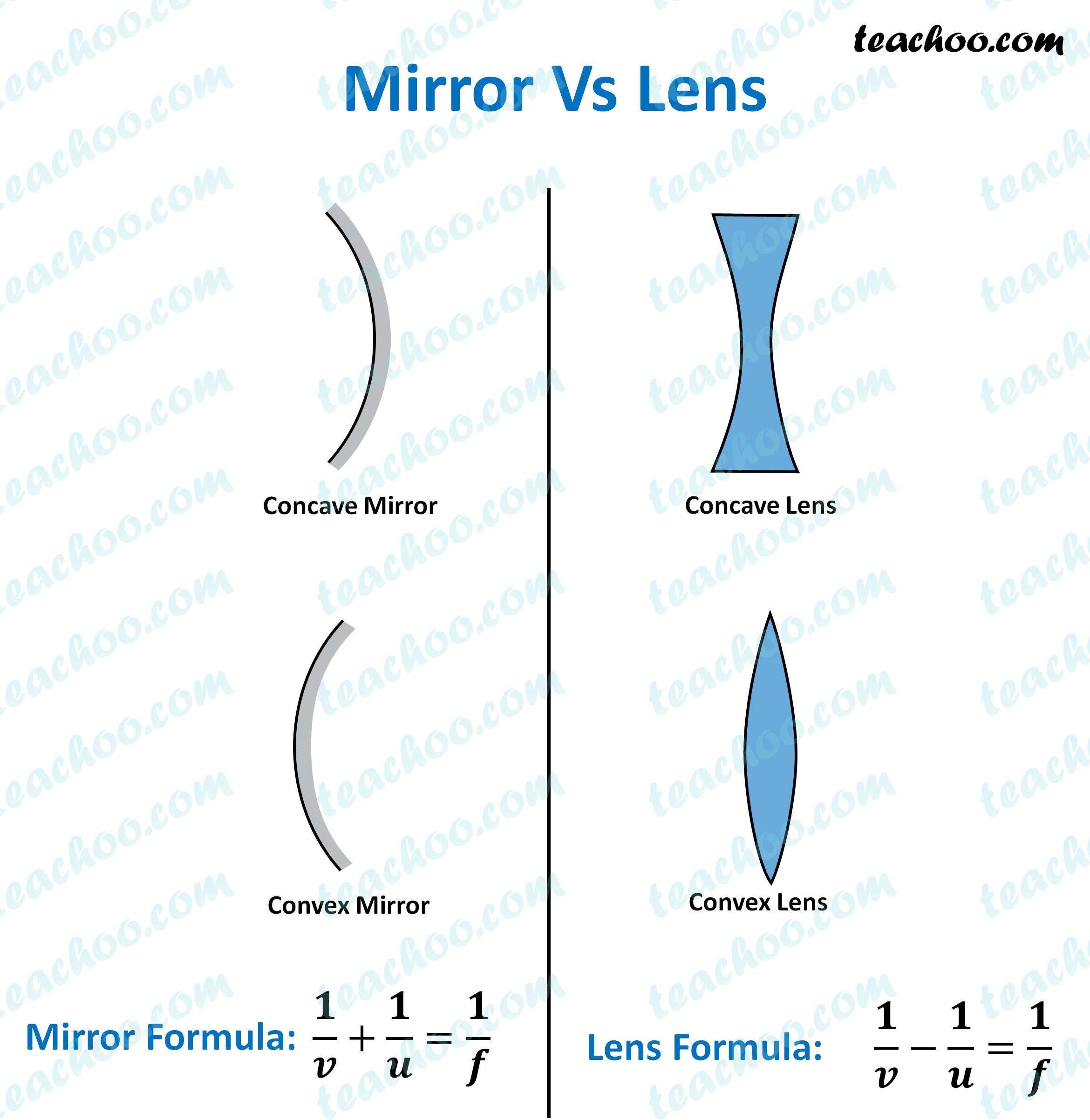Teachoo Questions

Class 10
Chapter 10 Class 10 - Light - Reflection and RefractionMirror Lens It has only one surface which reflects light. It has two surfaces, out of which one or both can refract light. It follows the laws of reflection. It follows the law of refraction. A mirror can be plane or spherical (concave or convex) A lens is usually curved from either one or both surfaces. Mirror formula is 1/f = 1/v + 1/u Lena formula is 1/f = 1/v - 1/u

Learn in your speed, with individual attention - Teachoo Maths 1-on-1 Class